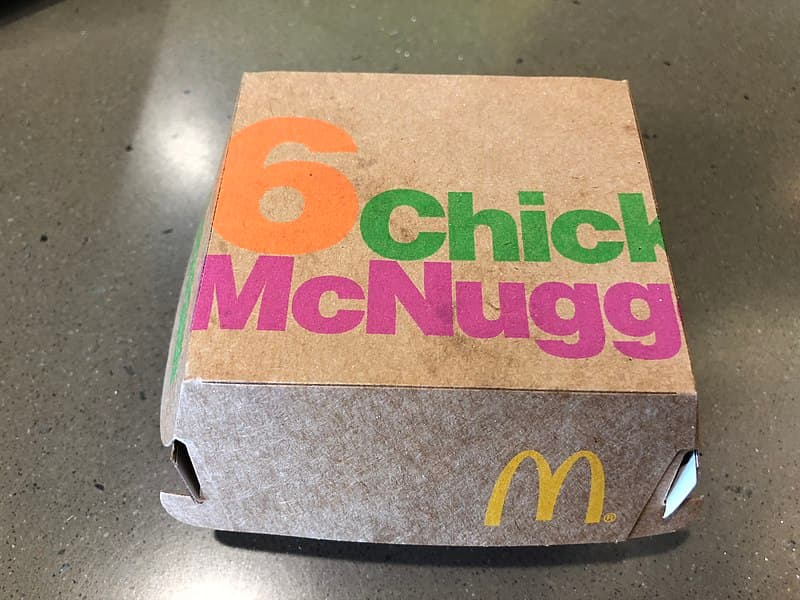# The Chicken McNugget Monoid

When McDonalds first introduced Chicken McNuggets, you could buy McNuggets in boxes of 6, 9, or 20. The Chicken McNugget problem is to determine which numbers of McNuggets you can and cannot buy. A number n is a McNugget number if it is possible to buy exactly that many McNuggets (using the original boxes).There are only finitely many non-McNugget numbers, and these are listed in OEIS sequence A065003.

Note that if you order eight boxes of 6 nuggets you get 48 nuggets, and so if you order more than eight boxes, in any combination, you get more than 48 nuggets. So the following program will compute all McNugget numbers less than 48.

```    ns = set()
for i in range(8):
for j in range(8):
for k in range(8):
n = 6*i + 9*j + 20*k
if n <= 48:

comp = set(range(48)) - ns
```

The non-McNugget numbers less than 48 are stored in `comp`, the set complement of `ns`. These numbers are

1, 2, 3, 4, 5, 7, 8, 10, 11, 13, 14, 16, 17, 19, 22, 23, 25, 28, 31, 34, 37, 43.

It turns out 48 and 49 are also McNugget numbers. You can verify this by changing “48” to “50” in the code above and looking at `ns`. This means that 44, 45, 46, 47, 48, and 49, a run of 6 consecutive numbers are all McNugget numbers, and so you can add boxes of 6 to these numbers to get any greater number. This shows that 43 is the largest non-McNugget number.

The set of McNugget numbers is contains 0, my favorite number of McNuggets, and is closed under addition, and so it forms a monoid.

Update: The next post generalizes this one, giving a little theory and more general code.

Source: Factoring in the Chicken McNugget monoid. arXiv:1709.01606